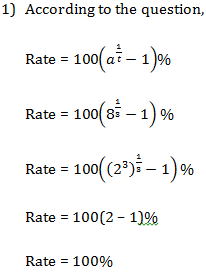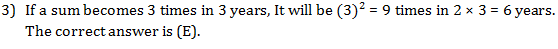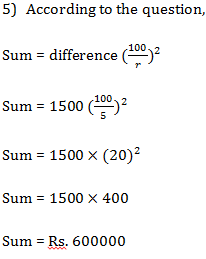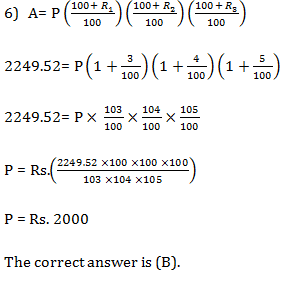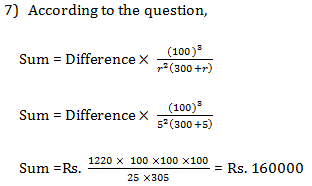# Simple and Compound Interest : 10 Important Questions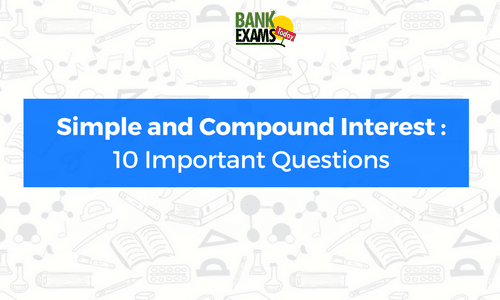1. At what percentage on compound interest will a sum of money become 8 times in 3 years?
(a) 300%
(b) 200%
(c) 100%
(d) 50%
(e) 20%

2. If the compound interest on a certain sum for two years at 3% be Rs. 10150. What would be the simple interest?
(a) Rs. 10000
(b) Rs. 12000
(c) Rs. 15000
(d) Rs. 20000
(e) Rs. 21000

3. A sum of money at compound interest amounts to thrice itself in three years. in how many years will it be 9 times itself?
(a) 2 years
(b) 9 years
(c) 3 years
(d) 12 years
(e) 6 years

4. There is 80% increase in an amount in 8 years at simple interest. What will be the compound interest of Rs.14,000 after 3 years at the same rate?
(a) Rs. 4236
(b) Rs. 4634
(c) Rs. 4422
(d) Rs. 4362
(e) Rs. 4262

5. The difference between the compound interest and the simple interest on a certain sum of money 5% per annum for 2 years is Rs. 1500. What is the sum?
(a) Rs. 150000
(b) Rs. 200000
(c) Rs. 450000
(d) Rs. 600000
(e) Rs. 620000

6. What is the sum of money at compound interest will amount to Rs. 2249.52 in 3 years, If the rate of interest is 3% for the first year, 4% for the second year and 5% for the third year?
(a) Rs. 1500
(b) Rs. 2000
(c) Rs. 4500
(d) Rs. 6000
(e) Rs. 6100

7. If the difference between the compound interest and the simple interest on a certain sum of money at 5% per annum for 3 years is Rs. 1220. What is the sum?
(a) Rs. 150000
(b) Rs. 200000
(c) Rs. 250000
(d) Rs. 160000
(e) Rs. 110000

8. The difference between compound interest and Simple interest on an amount of Rs. 15,000 for 2 years is Rs. 96. What is the rate of interest per annum?
(a) 6%
(b) 8%
(c) 12%
(d) 15%
(e) 14%

9. There is 60% increase in an amount in 6 years at simple interest. What will be the compound interest of Rs. 12,000 after 3 years at the same rate?
(a) Rs. 3972
(b) Rs. 3465
(c) Rs. 3564
(d) Rs. 3729
(e) Rs. 3529

10. The difference between simple interest and compound interest on Rs. 900 for one year at 10% per annum reckoned half yearly is:
(a) Rs. 2.15
(b) Rs. 2.25
(c) Rs. 2.35
(d) Rs. 1.15
(e) Rs. 2.15

Go through Simple and Compound Interest Tricks here